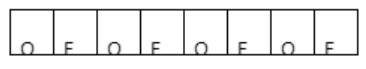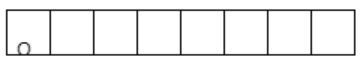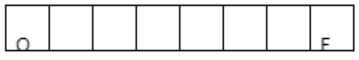QuestionAnswers

# How many different words can be formed with the letters of the word ORDINATE so that(i) The vowels occupy odd places(ii) Beginning with O(iii) Beginning with O and ending with E.Verified
145.5k+ views
Hint: For the first case, get the arrangements of vowel letters and consonants at odd and even places respectively, hence, get the total number of words using them. For the second case fix O at the first position and for third case fix O at first position and E at last. Now, get permutations of remaining letters to get the answer.

Complete step by step solution:
We have the word ‘ORDINATE’ which consists of letters O, R, D, I, N, A, T, E.
Here all the letters are different.
(i) The vowels occupy odd places. ORDINATE is an 8 letter word, so there will be 4 odd places and 4 even places.
Now, vowels in the given word can be given as O, I, A, E.\begin{align} & \text{O}\to \text{odd places} \\ & \text{E}\to \text{evenplaces} \\ \end{align}
Other letters except vowels in the given word are R, D, N, T.
As we can observe that there are 4 odd places and 4 vowels, so they will occupy each place once and we can arrange them by 4! as well. And similarly, we can arrange other letters (consonants) which will occupy even places by 4! as well, because there are 4 other letters except vowels and 4 even places.
Hence, the total words of all these kinds can be given by $4!\times 4!$.
Hence, the number of words where vowels occupy odd places are 576.
(ii) Beginning with 0.
Here, we need to find the number of words which are beginning with 0. So, let us fix ‘O’ at the starting of the given word ORDINATE, leaving 7 positions as empty.So, we have 7 letters R, D, I, N, A, T, E for the arrangement and formation of words.
Hence, the number of arrangements of 7 different letters is given as 7!.
So, number of words beginning with ‘o’
From ORDINATE can be given as 7!=5040.
(iii) Beginning with O and ending with E.
Here, we need to find the number of words starting with o and ending with E from ORDINATE. So, we can fix O and E in the beginning and last respectively, we have 6 positions as empty.So, now we have R, D, I, N, A, T for arrangement and calculating the total number of words for the required condition.
Hence, permutations of 6 letters can be given 6! (all different).
So, the number of words beginning with O and ending with E is 6! = 720.

Note: Here, we do not have any same letters with the word ORDINATE. If same letters are involved then permutations of them will be given by formula $\dfrac{n!}{{{r}_{1}}!{{r}_{2}}!{{r}_{3}}!............{{r}_{p}}!}$.
Here${{r}_{1}}{{r}_{2}}{{r}_{3}}......{{r}_{p}}$ are number of letters of same kind. So, take care of it as well.
Don’t count the number of vowels and consonants carelessly to not miss any cases for the first part.
Always try to solve these kinds of problems with the help of boxes to get the concepts of the problem. It makes the problem very flexible and easy. So, take care of it.